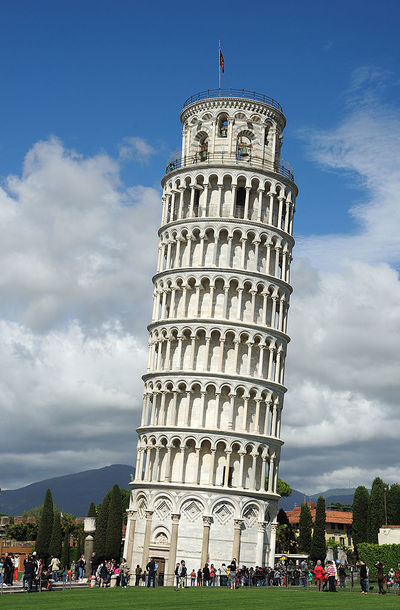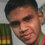# Do you think Galileo is correct?In the Laws of Gravity by Galileo, we say that every object take equal time to fall if there's no air resistance. We always ignore that The Earth will also have acceleration due to that object also ! So, if we don't ignore that concept, Are Galileo's Laws of Gravity correct? Think once again, can you explain your logic behind your opinion?

Traditionally, $g=\frac{\text{GM}}{r^2}$ Where, M=Mass of earth r=Distance between the object and the center of the earth

But in reality, earth will also have the acceleration due to the object of mass m. In that case, resultant acceleration, $g=g_o+g_e$ Here, $g_e$= Acceleration due to earth (for the object) And, $g_o$= Acceleration due to object (for the earth!) So, $g_o=\frac{\text{Gm}}{r^2}$ $g_e=\frac{\text{GM}}{r^2}$

Then, assume the earth is still. So, Relative (Actual) Acceleration of the object $g=g_o+g_e$ $=\frac{\text{G}}{r^2}\text{(M+m)}$

The equation says that Heavy objects have greater relative acceleration compared to lighter objects. So, heavy objects falls earlier !

So, in reality, in actuality, Galileo's Laws aren't perfect!

But most of the time we ignore acceleration of the earth (Acceleration due to object) , which makes calculations simpler!5 years, 4 months ago

This discussion board is a place to discuss our Daily Challenges and the math and science related to those challenges. Explanations are more than just a solution — they should explain the steps and thinking strategies that you used to obtain the solution. Comments should further the discussion of math and science.

When posting on Brilliant:

• Use the emojis to react to an explanation, whether you're congratulating a job well done , or just really confused .
• Ask specific questions about the challenge or the steps in somebody's explanation. Well-posed questions can add a lot to the discussion, but posting "I don't understand!" doesn't help anyone.
• Try to contribute something new to the discussion, whether it is an extension, generalization or other idea related to the challenge.

MarkdownAppears as
*italics* or _italics_ italics
**bold** or __bold__ bold
- bulleted- list
• bulleted
• list
1. numbered2. list
1. numbered
2. list
Note: you must add a full line of space before and after lists for them to show up correctly
paragraph 1paragraph 2

paragraph 1

paragraph 2

[example link](https://brilliant.org)example link
> This is a quote
This is a quote
    # I indented these lines
# 4 spaces, and now they show
# up as a code block.

print "hello world"
# I indented these lines
# 4 spaces, and now they show
# up as a code block.

print "hello world"
MathAppears as
Remember to wrap math in $$ ... $$ or $ ... $ to ensure proper formatting.
2 \times 3 $2 \times 3$
2^{34} $2^{34}$
a_{i-1} $a_{i-1}$
\frac{2}{3} $\frac{2}{3}$
\sqrt{2} $\sqrt{2}$
\sum_{i=1}^3 $\sum_{i=1}^3$
\sin \theta $\sin \theta$
\boxed{123} $\boxed{123}$

Sort by:

Galileo was (is?) correct. It is easy to calculate the acceleration of a mass m1 adjacent to another mass m2. Fix the first mass as 1000kg and the second mass as 100kg at a distance of 1 metre. Now do the calculation again with m1 the same but m2 halved. The acceleration of m2 is the same, it is the acceleration of m1 that changes. The reason is pretty obvious when you look at the maths. When you change m2 the force of attraction changes in direct proportion to the mass. So halve the mass m2, the force halves. The acceleration is proportional to the force and inversely proportional to the mass. Halve both and the acceleration stays the same. QED.

- 2 months, 2 weeks ago

I agree with Arifur's idea..he wants to say that both earth and the object attract each other..the object's acceleration depends on the earth's mass and the earth's acceleration on the object's mass.when we look from the earth at the object falling, what we are observing is its relative acceleration w.r.t. the earth frame which in turn depends on both by the relationship that Arifur has mentioned in his note.

Exactly. I wish we could have Galileo alive to correct him!

Galileo made only some experimental observations without knowing what law works behind.. and the error due to the earth's acceleration is so negligible that it can be hardly measured by experiments..further when someone is dropping a body at one location of the earth's surface..there may be bodies which are being dropped (or falling) at various different location..which may even nullify the first body's effect on the earth..so experiments can hardly detect it.

Yeah, the object needs to be $6\times 10^{22}kg$ to increase the g by only 1%. Most probably this isn't measurable anyway. And when there are thousands of objects being dropped every moment, nullification is so common. We can't even witness such difference.

Yup! That's what I am saying.

We were taught in the school that irrespective of the weight, when two objects of different weight are thrown at the same time from the same height in an ideal resistance-less atmosphere both would reach the earth at one and the same time as per Galileo's Law.I had my own doubt though I could not disprove this Galileo Law and felt that heavier object would reach the earth slightly earlier than the lighter one, even though the difference in time could be too small to be taken into account. Arifur Rahman's proof as above seems to vindicate what I had doubted. One formula in Physics says F=ma, where F is the force and m and a are mass and acceleration respectively of the objects. That means Force (F) generated is a function of mass (m) and acceleration (a). Heavier the mass or more the acceleration or both more would be the Force exerted. Obviously though the acceleration remains the same and the mass varies or if mass remains the same and acceleration varies, the heavier object and the object with higher acceleration would be acted upon by more force than the lighter one/or with less acceleration and hence the heavier object with more acceleration would move a bit faster and reach the earth earlier than the lighter one howsoever smaaaaaal the difference in the force is. Further, every object in the Universe attracts the other objects even if the force of attraction is too small to be felt. As such, the falling objects attract the earth and so also the the earth the falling objects. This force also affects the motion of the falling objects. The Heavier will be attracted by more force and the lighter one with less force. This also makes their motion slightly different one from each other. In the end, I am inclined to agree with Rahman when he says when we ignore something because of its negligible value does not mean it does not exist. Hence we may say that the Galileo Law, though almost nearest to the actual finding, it has some minor difference which we have been ignoring. I invite Arifur Rahman to comment on what I have stated and add his views to this.

- 5 years, 3 months ago

Very thoughtful explanation. Its nearly correct, but not totally accurate. Yes, we don't need to consider earth's acceleration. But if you want pinpoint correct answer, you will have to do a little bit more.

Thank you, Venkatesh Patil for your nice writeup which made this note precise.

BTW, If you're not in the Brilliant Lounge , join today. We will be happy to have you onboard.

- 5 years, 3 months ago

@Arifur Rahman This equation is very hard to understand:

$g = g_o+g_e$

What object is supposed to have the acceleration $g_o+g_e$ in your situation?

Staff - 5 years, 4 months ago

Simple. And its already mentioned in the Note . Assume earth to be still, then the object is coming towards the earth with acceleration, g. Or, if you prefer so, assume the object isn't moving, then the earth is reaching the object with acceleration, g .
Its just to make hideous calculations simplest.

So, if you don't like to use any of the above techniques, do your own equations. But whatever you do, you can't neglect Acceleration due to object this time.

Actually, its much more conceptual, rather than being analytical. Make your brain thinking and everything will be crystal clear.

- 5 years, 4 months ago

Oh, now I see what you are saying. If we hold the Earth, or the object still, then the acceleration of one of them relative to the other will happen at $g$. How massive does the object have to be before we can notice this effect?

Staff - 5 years, 4 months ago

I think Josh Silverman, who's a problemsetter of the "IPhOO 2014" , knows the answer, without anyone's help.

- 5 years, 4 months ago

I still doubt that. Where did the g_0 come from in the first place, then?

- 5 years, 4 months ago

That $g_o$ is the Acceleration due to the object , as its already mentioned. And this is what you neglect in your Galilean Equations.

- 5 years, 4 months ago

How massive would the object need to be to change its measured acceleration by say 1%?

Staff - 5 years, 4 months ago

A nice Brilliant problem.

Your question is a little ambiguous - 1% change in g - increase or decrease? Well, obviously its increase Then $g_2=g_1(1+.01)=1.01g_1$ In general, $g_1=\frac{\text{GM}}{r^2}$ Using proportionality concept, $\frac{g_2}{g_1}=\frac{\text{M}+m}{\text{M}}$ $\rightarrow 1.01\text{M}=\text{M+m}$ $\rightarrow m=0.01\text{M}$ You can take Mass of the earth, $\text{M}=6\times 10^{24} kg$ Then, $m=6\times 10^{22} kg$

Notice that, its quite a Huge mass. Which necessarily means we can neglect it.

But Neglecting something doesn't mean the thing doesn't EXISTS!.

- 5 years, 4 months ago

You're looking and searching here and there for a Reference Frame, right?

Well, make the universe your reference frame (though nothing in this holy Universe is stationery!) . You'd like some more calculations. You might've solved Train Collision type problems. Both trains decelerate to Stop a collision from a distance(linear, of course) d. What Reference Frame you use there? - The surroundings , to be more precise Earth is the reference frame there.

So, if the distance between Center of the earth and the Object is r You'll make, $r=\frac{1}{2}g_et^2+\frac{1}{2}g_ot^2$ $r=\frac{1}{2}(g_e+g_o)t^2$ I think its excess to explain how we came up here. And $(g_e+g_o)$ is that so called Relative Acceleration and you can find out millions of Reference Frames around Earth- Object Attraction

Its a bit surprising that Agnishom is asking this type of questions. Because your Physics Level is relatively higher that average explorers on Brilliant.

- 5 years, 4 months ago

If the earth is the frame of reference, are we not supposed to see it still?

- 5 years, 4 months ago

I mentioned The surroundings around earth and the object is that reference frame. If earth was the RF, then what I'm talking about!

- 5 years, 4 months ago

You cannot add up those accelerations. By Newton's Third Law, they are equal amd opposite, which is perfectly okay because we're seeing one of them from the earth's coordinates and the other from the object's reference frame. When you mean to add them up, what reference frame are you using?

If this is unclear with A, let's look at velocities. If A is moving towards B with speed 5, it should appear that B is also moving towards A with speed 5. So, would that mean A is approaching B with velocity 10?

- 5 years, 4 months ago

@Agnishom Chattopadhyay pls check out this note.

- 5 years, 4 months ago

Thanks for the mention. I find something fishy here.

- 5 years, 4 months ago

You find something fishy, Agnishom Chattopadhyay !

You could've found a better word for the comment. Its like, you're forcing the reality like Catholic Churches.

- 5 years, 4 months ago

I'm sorry you're offended by that word. I meant that your idea looks a bit wrong.

- 5 years, 4 months ago

Thank you. That's called frankly speaking, being friendly over the internet. You're appreciating.

- 5 years, 4 months ago